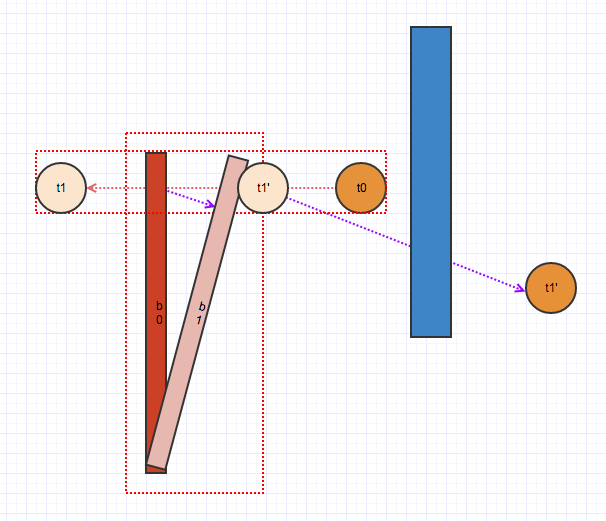# Unity Collision Detection

About three or four years ago, I was development my first indie game, and my main character have a gun, well if you know something about unity, you know what happened, the bullet go through enemy because of the tunnel effect because of the velocity is too large. At that time I know little about unity so my solution was just slow down the bullet.

However, there is a solution provided by Unity itself somehow I missed it, so I decided to write something about it.

In Rigidbody component, there is a setting of collision detection that you can choose one of four detection mode:

Mode Description
Discrete Use discrete collision detection against all other Colliders in the Scene . Other colliders will use discrete collision detection when testing for collision against it. Used for normal collisions (This is the default value).
Continuous Use Discrete collision detection against dynamic Colliders (with a Rigidbody) and sweep-based continuous collision detection against static Colliders (without a Rigidbody). Rigidbodies set to Continuous Dynamic will use continuous collision detection when testing for collision against this rigidbody. Other rigidbodies will use Discrete Collision detection. Used for objects which the Continuous Dynamic detection needs to collide with. (This has a big impact on physics performance, leave it set to Discrete, if you don’t have issues with collisions of fast objects)
Continuous Dynamic Use sweep-based continuous collision detection against GameOjects set to Continuous and Continuous Dynamic collision. It will also use continuous collision detection against static Colliders (without a Rigidbody). For all other colliders, it uses discrete collision detection. Used for fast moving objects.
Continuous Speculative Use speculative continuous collision detection against Rigidbodies and Colliders. This is also the only CCD mode that you can set kinematic bodies. This method tends to be less expensive than sweep-based continuous collision detection.

Before look into these mode, let’s review some collision algorithm. Consider a situation, we have a mess number of object in our space, and we want to report all object that intersect with others, if we compare every pair of objects, it will takes $O(n^2)$, not bad, but if we calculate it every frame, for Unity, it will be calculated in FixedUpdate which is called 50 times per second by default, it will be come a problem, so we would like to filter some object that’s unlikely to collide.

For broad phase of collision detection, we combine 3 methods:

• Simplify the collision mesh.
• Index the world space into some sort of data structure.
• Sweep and Prune.

## AABB-Box collision

AABB, stand for Axis align bounding box, is a technology that bounding object with the minimal rectangle or cuboid which edges parallel to the x, y and maybe z axis.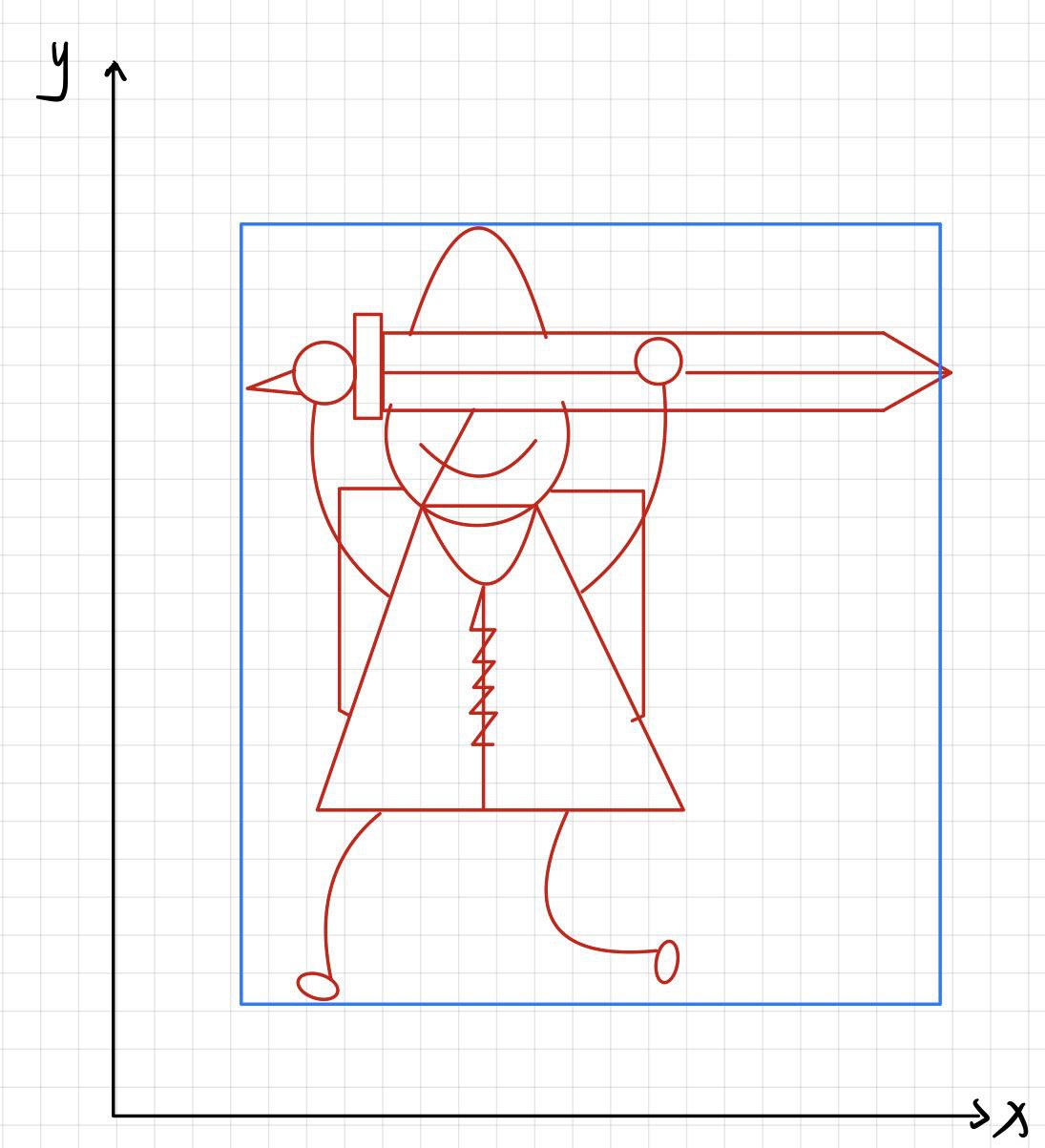By this simplification, we don’t care about the detail of object anymore and the collision detection algorithm will become very simple and fast: we just need to check whether these object’s projections overlap in the x, y and maybe z axes.

## Space division

After bounding objects, do we need to check each pair of object? If so, the time complexity wouldn’t improve much. We can construct the world space to some data structure which is easy for insert, delete and query, and we only check collision in a certain sub-area that contain more than one object. Well then the answer will be clear, we can use range-tree, kd-tree, quadtree, octree and many other solutions. Another problem we need to care about is the granularity of the space division.

## Sweep and Prune

Now suppose we found a sub-area that need to do collision check, by observation, two objects collide if and only if all of their projections to the axis have intersection, which means if two objects are not collide, their projects has no intersection in at least one axis. Since we are doing broad phase collision detection, we can just check intersection in only one axis, and that will be come an 1-D line intersection detection problem, which can be solved by sweep-line algorithm.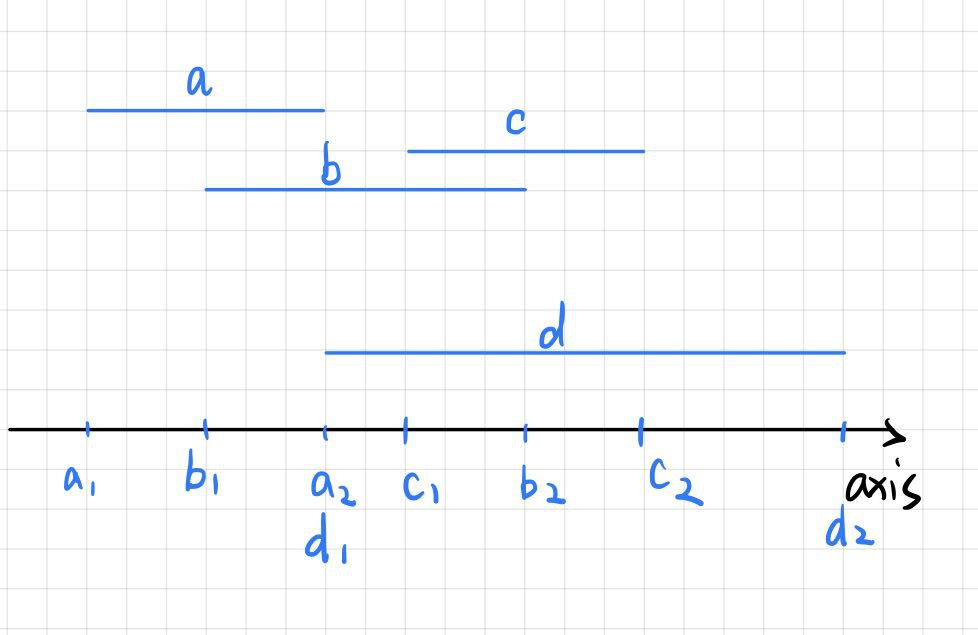So what about the time complexity? Well sweep-line algorithm takes $O(n)$ but the data need to be sorted, which takes another $O(n\log n)$, but according to the frame coherence, the relative position between objects wouldn’t change much in two adjacent frames, so we can consider that every sort algorithm is run under a good enough situation, and some algorithm for example insert sorting algorithm can be done in $O(n)$, so the overall time complexity is $O(n)$.

## Discrete

Discrete mode simply check collision in each frame by treat objects as static, it doesn’t consider any velocity or physics stuff, so if a wall is very thin and a object might pass through it in one frame.

## Continuous collision detection

This algorithm is equivalent to finding the limit of the movement of an object in unit time and judging its collision with other objects

### Sweep-based

Calculate the position of the object in next frame using the linear motion’s velocity, and check if there exists any object between these two position.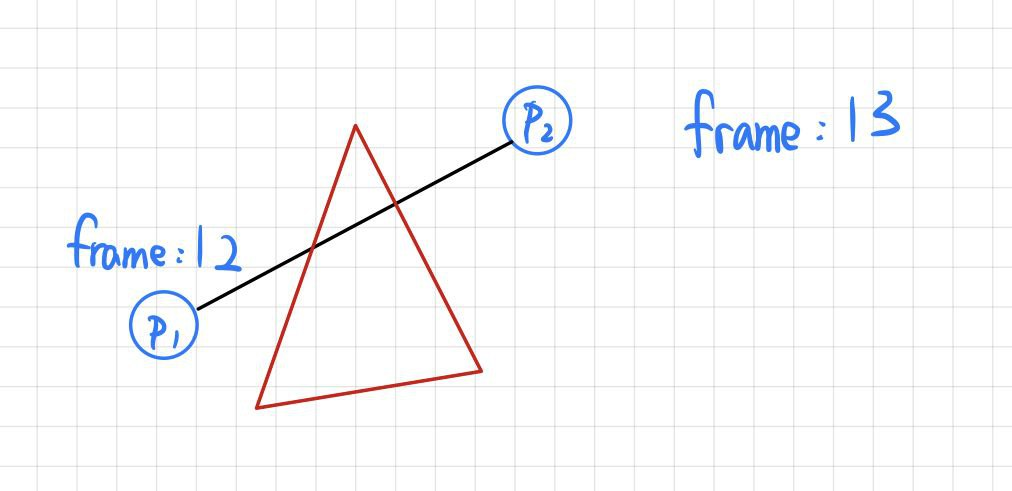However, this algorithm ignore the angular motion of the object and that might cause fellow problem:### Speculative

This algorithm includes two part:

• A new AABB-box bounding the object this frame and the object in the potential position in next frame, calculating with not only the linear motion but also the angular motion: (The red rectangle is the AABB-box).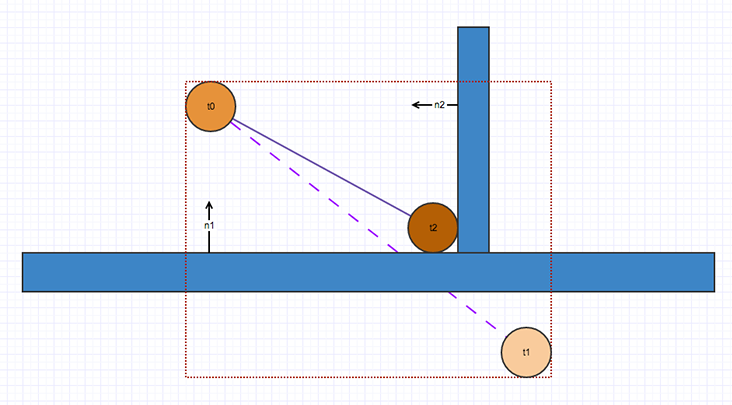• A solver that collect all potential contacts and makes sure that all contact constraints are satisfied. In image above, $n1$ and $n2$ are the force constrains.

However because potential contact are are calculate by the closet point algorithm (Can be done by divide and conquer, Delaney triangulation), and the implementation of this algorithm done by Unity is somehow inaccurate, which means the actual collision mesh will be bigger, it might cause ghost collision when it shouldn’t collide.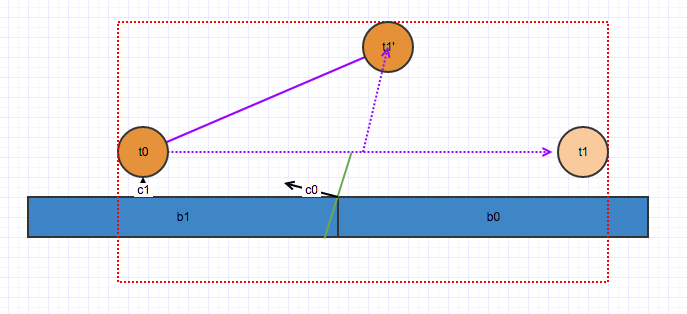Another problem (Gosh you got so many problem) is that because the solver only works while collision happen, and it only consider potential contacts it meet so far, if some object slams into this object, it might fly out of the AABB-box, and have no collision with objects on the path.